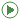## OpenIPSL.Tests.ThreePhase

Set of simple systems to test the functionality of three-phase and hybrid positive-sequence/three-phase interface

### Information

This package contains test systems and examples that can be used to understand how models from the ThreePhase package should be used, and what results should be expected.

Extends from Modelica.Icons.ExamplesPackage (Icon for packages containing runnable examples).

### Package Content

Name DescriptionIEEE4 Initial conditions (power-flow) calculation of IEEE4 bus system, in full three-phase formulationIEEE4_MonoTri Initial conditions (power-flow) calculation of IEEE4 bus system, in hybrid positive-sequence/three-phase formulationIEEE13 Initial conditions (power-flow) calculation of IEEE4 bus system, in full three-phase formulation

##OpenIPSL.Tests.ThreePhase.IEEE4

Initial conditions (power-flow) calculation of IEEE4 bus system, in full three-phase formulation

### Information

This test system aims to show how a very small three-phase system should be assembled for simulation. Its results should be compared to the example IEEE4_MonoTri, in order to show what to expect from the hybrid transformer. Here, all buses are modeled as three-phase buses.

This example is not a dynamic system, and therefore the voltages and angles from all buses are static during the simulation. The simulation just calculates the initialization variables, which are the solution for the three-phase power flow. The results can be verified against any tool that calculates a three-phase power-flow.

Extends from Modelica.Icons.Example (Icon for runnable examples).

##OpenIPSL.Tests.ThreePhase.IEEE4_MonoTri

Initial conditions (power-flow) calculation of IEEE4 bus system, in hybrid positive-sequence/three-phase formulation

### Information

This test system aims to show how the hybrid positive-sequence/three-phase transformer should be used for simulation. Its results should be compared to the example IEEE4, in order to show what to expect from the hybrid transformer. The MonoTri device interconnects to parts of the system which are modeled with different formulations. The first two buses are positive-sequence only while buses three and four are three-phase buses.

This example, however, is not a dynamic system, and therefore the voltages and angles from all buses are static during the simulation. The simulation just calculates the initialization variables, which are the solution for the hybrid positive-sequence/three-phase power flow. The results can be verified against any tool that calculates a hybrid positive-sequence/three-phase power-flow.

Extends from Modelica.Icons.Example (Icon for runnable examples).

##OpenIPSL.Tests.ThreePhase.IEEE13

Initial conditions (power-flow) calculation of IEEE4 bus system, in full three-phase formulation

### Information

This test system aims to show how a three-phase system should be assembled for simulation. In this example, there are many different models being used, like two-phase lines, buses and loads.

This example is not a dynamic system, and therefore the voltages and angles from all buses are static during the simulation. The simulation just calculates the initialization variables, which are the solution for the three-phase power flow. The results can be verified against any tool that calculates a three-phase power-flow.

Extends from Modelica.Icons.Example (Icon for runnable examples).

Automatically generated Mon Oct 10 14:35:56 2022.